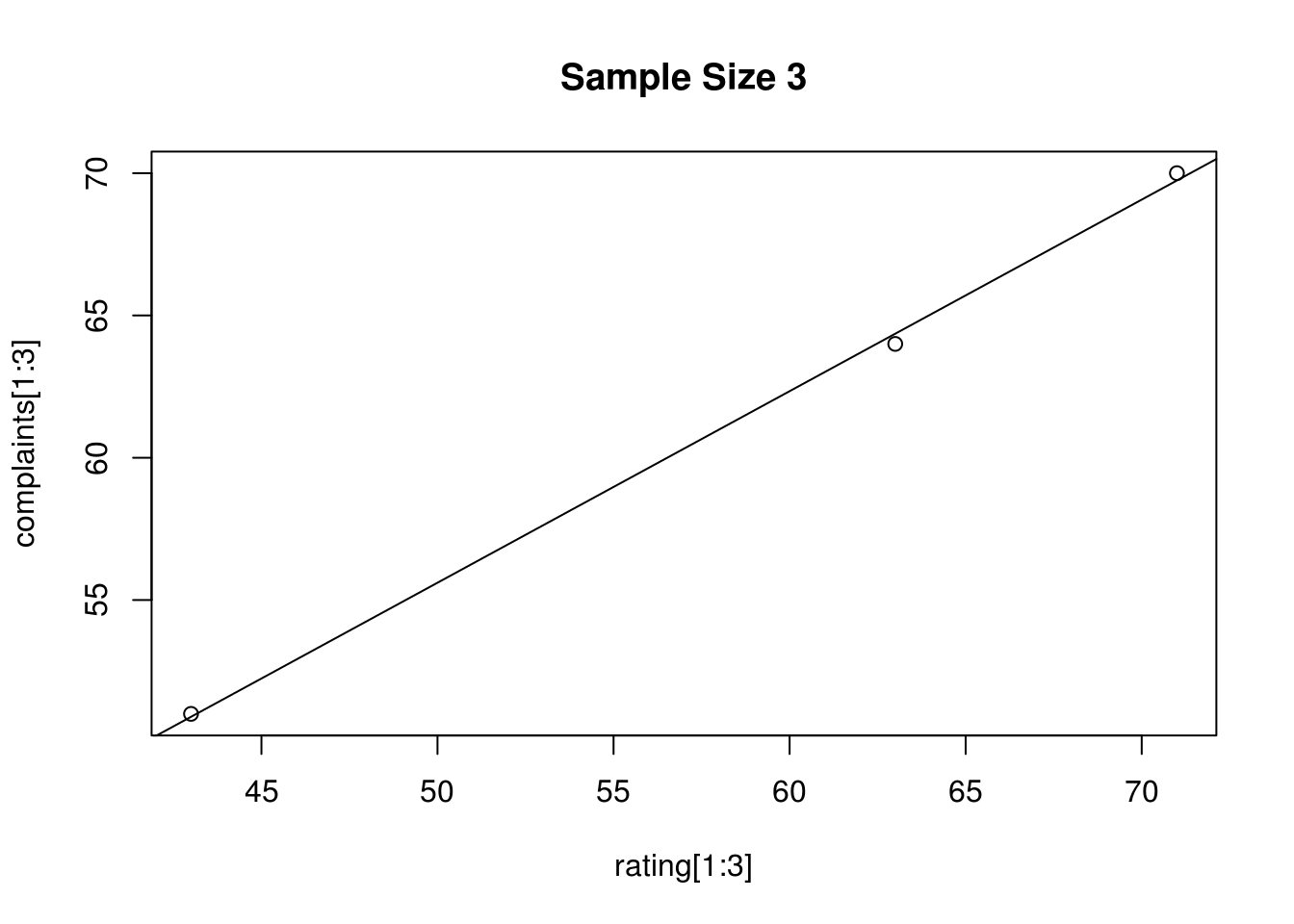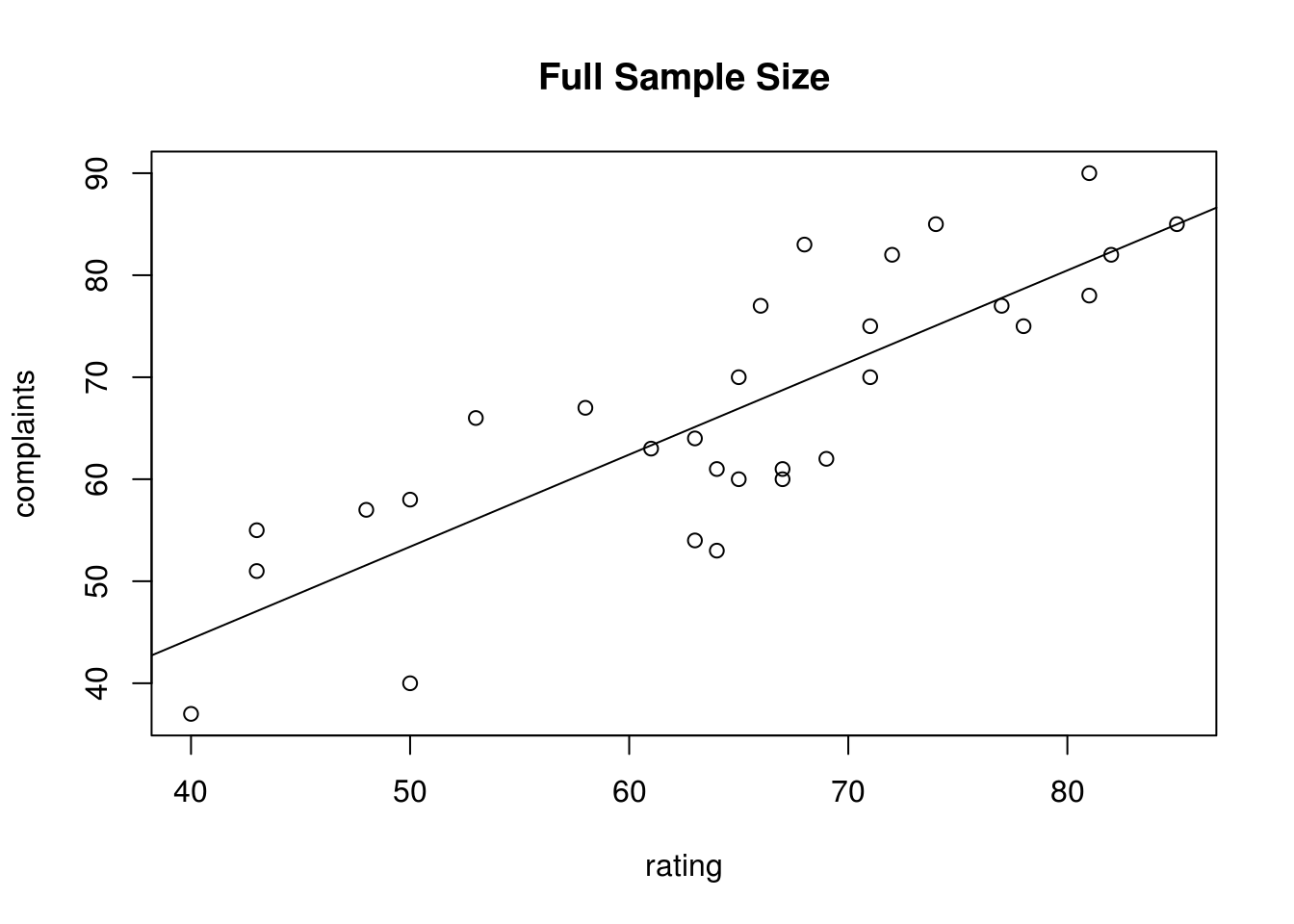# High Dimensionality Regression

There are times when least squares regression is not able to provide accurate predictions or explanation in an object. One example in which least scaresregression struggles with a small sample size. By small, we mean when the total number of variables is greater than the sample size. Another term for this is high dimensions which means more variables than examples in the dataset

This post will explain the consequences of what happens when high dimensions is a problem and also how to address the problem.

Inaccurate measurements

One problem with high dimensions in regression is that the results for the various metrics are overfitted to the data. Below is an example using the “attitude” dataset. There are 2 variables and 3 examples for developing a model. This is not strictly high dimensions but it is an example of a small sample size.

``````data("attitude")
reg1 <- lm(complaints[1:3]~rating[1:3],data=attitude[1:3])
summary(reg1)``````
``````##
## Call:
## lm(formula = complaints[1:3] ~ rating[1:3], data = attitude[1:3])
##
## Residuals:
##       1       2       3
##  0.1026 -0.3590  0.2564
##
## Coefficients:
##             Estimate Std. Error t value Pr(>|t|)
## (Intercept) 21.95513    1.33598   16.43   0.0387 *
## rating[1:3]  0.67308    0.02221   30.31   0.0210 *
## ---
## Signif. codes:  0 '***' 0.001 '**' 0.01 '*' 0.05 '.' 0.1 ' ' 1
##
## Residual standard error: 0.4529 on 1 degrees of freedom
## Multiple R-squared:  0.9989, Adjusted R-squared:  0.9978
## F-statistic: 918.7 on 1 and 1 DF,  p-value: 0.021``````

With only 3 data points the fit is perfect. You can also examine the mean squared error of the model. Below is a function for this followed by the results

``````mse <- function(sm){
mean(sm\$residuals^2)}
mse(reg1)``````
``##  0.06837607``

Almost no error. Lastly, let’s look at a visual of the model

``````with(attitude[1:3],plot(complaints[1:3]~ rating[1:3]))
title(main = "Sample Size 3")
abline(lm(complaints[1:3]~rating[1:3],data = attitude))``````You can see that the regression line goes almost perfectly through each data point. If we tried to use this model on the test set in a real data science problem there would be a huge amount of bias. Now we will rerun the analysis this time with the full sample.

``````reg2<- lm(complaints~rating,data=attitude)
summary(reg2)``````
``````##
## Call:
## lm(formula = complaints ~ rating, data = attitude)
##
## Residuals:
##      Min       1Q   Median       3Q      Max
## -13.3880  -6.4553  -0.2997   6.1462  13.3603
##
## Coefficients:
##             Estimate Std. Error t value Pr(>|t|)
## (Intercept)   8.2445     7.6706   1.075    0.292
## rating        0.9029     0.1167   7.737 1.99e-08 ***
## ---
## Signif. codes:  0 '***' 0.001 '**' 0.01 '*' 0.05 '.' 0.1 ' ' 1
##
## Residual standard error: 7.65 on 28 degrees of freedom
## Multiple R-squared:  0.6813, Adjusted R-squared:  0.6699
## F-statistic: 59.86 on 1 and 28 DF,  p-value: 1.988e-08``````

You can clearly see a huge reduction in the r-square from .99 to .68. Next, is the mean-square error

``mse(reg2)``
``##  54.61425``

The error has increased a great deal. Lastly, we fit the regression line

``````with(attitude,plot(complaints~ rating))
title(main = "Full Sample Size")
abline(lm(complaints~rating,data = attitude))``````Naturally, the second model is more likely to perform better with a test set. The problem is that least squares regression is too flexible when the number of features is greater than or equal to the number of examples in a dataset.

What to Do?

If least squares regression must be used. One solution to overcoming high dimensionality is to use some form of regularization regression such as ridge, lasso, or elastic net. Any of these regularization approaches will help to reduce the number of variables or dimensions in the final model through the use of shrinkage.

However, keep in mind that no matter what you do as the number of dimensions increases so does the r-square even if the variable is useless. This is known as the curse of dimensionality. Again, regularization can help with this.

Remember that with a large number of dimensions there are normally several equally acceptable models. To determine which is most useful depends on understanding the problem and context of the study.

Conclusion

With the ability to collect huge amounts of data has led to the growing problem of high dimensionality. One there are more features than examples it can lead to statistical errors. However, regularization is one tool for dealing with this problem.